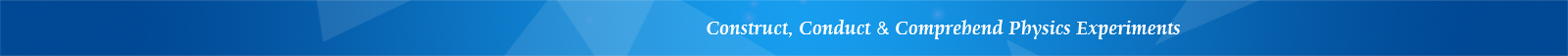## LEMI-18 Rotational Moment of Inertia Apparatus

Features

• Optical rail for simple adjustments

• High accuracy

• Affordable

Introduction

Moment of inertia is a physical quantity for the representation of the inertial amount of a rotational object. It is dependent on the mass distribution and the shaft position of the rigid body.

This apparatus uses the three-string pendulum method to determine the moment of inertia of a rigid body. A photoelectric sensor combined with a time counting device is used to measure the torsional oscillation period of a hanging plate. Through the experiments, students can understand the physical principle of the moment of inertia and learn the measurement method with factors related to the amount of moment of inertia.

Using this apparatus, the following experiments can be conducted:

1. Measure the moment of inertia using the three-string pendulum method

2. Measure the moment of inertia of two objects of same mass but with different mass distribution

3. Verify the parallel axis theorem of moment of inertia